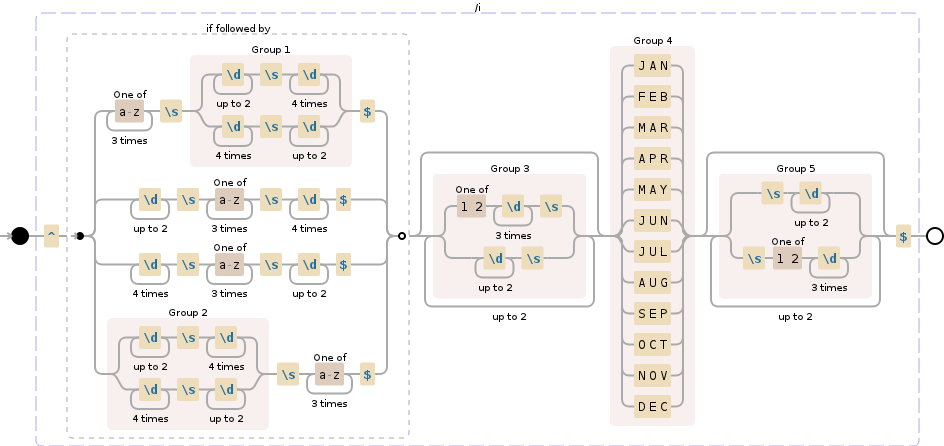Check valid date combinations using Regex?

A user can enter a few valid date combinations into an input :

Examples : ( all combinations are allowed 3!=6)

``````feb 7 2012
feb 07 2012
7 feb 2012
2012 7 feb
...
``````

All are valid dates.

I have also managed to create a regex which check it :

`/^(?=.*\b(JAN|FEB|MAR|APR|MAY|JUN|JUL|AUG|SEP|OCT|NOV|DEC)\b)(?=.*(\b[1‌2]\d{3}\b))(?=.*\b(0[1-9]|\d|3)\b).*\$/i`

Which means :

• `(?=.*\b(JAN|FEB|MAR|APR|MAY|JUN|JUL|AUG|SEP|OCT|NOV|DEC)\b)` : I'm expecting one of those values at the future.
• `(?=.*(\b[1‌2]\d{3}\b))` : I'm expecting year : 1xxx or 2xxx
• `(?=.*\b(0[1-9]|\d|3)\b)` I'm expecting day : 0x or 1x or 2x or 3x

All are OK.

So where is the problem ?

My regex also matches an invalid solutions which includes a valid solution :

IE : `feb 13 2012 4` will also pass.

``````Console :

/^(?=.*\b(JAN|FEB|MAR|APR|MAY|JUN|JUL|AUG|SEP|OCT|NOV|DEC)\b)(?=.*(\b[1‌2]\d{3}\b))(?=.*\b(0[1-9]|\d|3)\b).*\$/i.test('feb 13 2012 4') //true
``````

Question :

How can I enhance my regex in order to find a strict match ?

p.s. Checking `new Date(xxx)` is also a solution , but I'm looking for regex solution.(to improve my regex skills).

• what code are you using to execute your expression match? – norlesh Jan 6 '14 at 8:14
• @norlesh Just using console : `/^(?=.*\b(JAN|FEB|MAR|APR|MAY|JUN|JUL|AUG|SEP|OCT|NOV|DEC)\b)(?=.*(\b[1‌2]\d{3}\b))(?=.*\b(0[1-9]|\d|3)\b).*\$/i.test('feb 13 2012 4')` – Royi Namir Jan 6 '14 at 8:14
• Instead of trying to mash all the different formats into one regexp, make a separate regexp for each format, and combine them with alternation. – Barmar Jan 6 '14 at 8:15
• @Barmar would it be rude to ask for a sample ? :-) – Royi Namir Jan 6 '14 at 8:16
• what is the last `.*` doing there before `\$`? I would expect that is what is letting the trailing stuff through, but I can't get the expression to let anything through without it. – norlesh Jan 6 '14 at 8:43

Create one look-forward `(?=...)` group at the beginning, then optional groups for digits before or after the month block. The regex below works for all of your examples (see the unit tests at the linked page). Edit: it now matches all 3!=6 allowed combinations, but no impermissible combinations like `2 feb 1978 4`.

``````/^(?=[a-z]{3}\s(\d{1,2}\s\d{4}|\d{4}\s\d{1,2})\$|\d{1,2}\s[a-z]{3}\s\d{4}\$|\d{4}\s[a-z]{3}\s\d{1,2}\$|(\d{1,2}\s\d{4}|\d{4}\s\d{1,2})\s[a-z]{3}\$)(\d{3}\s|\d{1,2}\s){0,2}(JAN|FEB|MAR|APR|MAY|JUN|JUL|AUG|SEP|OCT|NOV|DEC)(\s\d{1,2}|\s\d{3}){0,2}\$/i
``````Debuggex Demo

Explanation:

• The entire expression must look like one of the following:
• Three of `[a-z]` followed by a day, then a year
• Three of `[a-z]` followed by a year, then a day
• A day, three of `[a-z]`, and a year
• A year, three of `[a-z]`, and a day
• A day, a year, then three of `[a-z]`
• A year, a day, then three of `[a-z]`
• Optionally match one or both of the number groups
• Match the three-letter month code
• Optionally match one or both of the number groups
• Visualization by Debuggex ;) – sp00m Jan 6 '14 at 8:39
• your solution also matches `2 1978 feb 3` – Royi Namir Jan 6 '14 at 8:42
• @sp00m Oops; forgot to paste the link the first time. – Ed Cottrell Jan 6 '14 at 8:43
• @EdCottrell `false /^(?=[a-z]{3}\s\d*\s\d*\$|\d*\s[a-z]{3}\s\d*\$|\d*\s\d*\s[a-z]{3}\$)(\d{4}\s)?(\d{1,2}\s)?(JAN|FEB|MAR|APR|MAY|JUN|JUL|AUG|SEP|OCT|NOV|DEC)(\s\d{1,2})?(\s\d{2}|\s\d{3})?\$/i.test('2 1978 feb')` return false – Royi Namir Jan 6 '14 at 8:44
• @RoyiNamir No, it doesn't match `2 1978 feb 3`; try it. You can see at the Debuggex link above that it doesn't match that pattern. As for `2 1978 feb`, that isn't one of the patterns you originally identified as one you want to match (and isn't a standard date format anywhere, as far as I know). This is easily fixed, if you want to match it. – Ed Cottrell Jan 6 '14 at 8:46

Write a regexp for each format:

• RE1 - recognizes `MON DD YYYY` = `(?:JAN|FEB|MAR|APR|MAY|JUN|JUL|AUG|SEP|OCT|NOV|DEC)\s+(?:0?[1-9]|\d|3)\s+\d{3}`
• RE2 - recognizes `DD MON YYYY` = `(?:0?[1-9]|\d|3)\s+(?:JAN|FEB|MAR|APR|MAY|JUN|JUL|AUG|SEP|OCT|NOV|DEC)\s+\d{3}`
• RE3 - recognizes `YYYY DD MON` = `\d{3}\s+(?:0?[1-9]|\d|3)\s+(?:JAN|FEB|MAR|APR|MAY|JUN|JUL|AUG|SEP|OCT|NOV|DEC)`

Then combine them:

``````/^(?:RE1|RE2|RE3)\$/i
``````
• there should be (according to your solution 3! regexs ( 3!=6).... – Royi Namir Jan 6 '14 at 8:37
• Only if you want to allow every permutation. My solution only allows the 3 permutations you listed, it doesn't allow `MON YYYY DD`, for instance. – Barmar Jan 6 '14 at 8:40